Hydrogen Atom Ground State in a E-field, the Stark Effect.

We have solved the Hydrogen problem with the following Hamiltonian.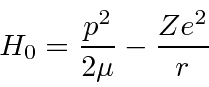Now we want to find the correction to that solution if an Electric field is applied to the atom. We choose the axes so that the Electric field is in the z direction. The perturbtion is then.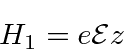It is typically a small perturbation. For non-degenerate states, the first order correction to the energy is zero because the expectation value of z is an odd function.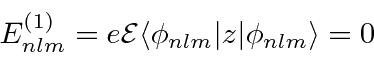We therefore need to calculate the second order correction. This involves a sum over all the other states.We need to compute all the matrix elements of z between the ground state and the other Hydrogen states.We can do the angular integral by converting theterm into a spherical harmonic.The we can just use the orthonormality of the spherical harmonics to do the angular integral, leaving us with a radial integral to do.The radial part of the integral can be done with some work, yielding.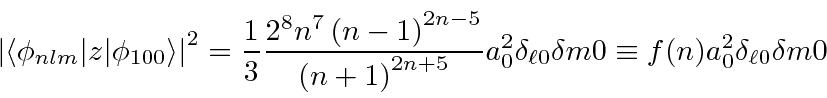We put this back into the sum. The Kronecker deltas eliminate the sums overand. We write the energy denominators in terms of the Bohr radius.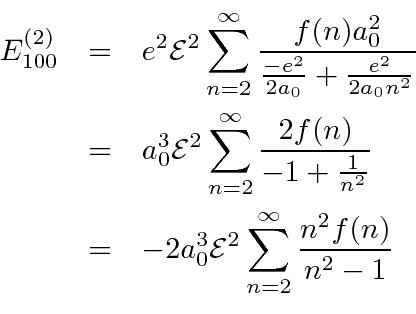This is all a little dissatisfying because we had to insert the general formula for the radial integral and it just goes into a nasty sum. In fact, we could just start with the first few states to get a good idea of the size of the effect. The result comes out to be.The first two terms of the sum get us pretty close to the right answer. We could have just done those radial integrals.

Now we compute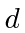, the electric dipole moment of the atom which is induced by the electric field.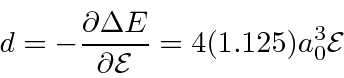The dipole moment is proportional to the Electric field, indicating that it is induced. The E field induces the dipole moment, then the dipole moment interacts with the E field causing a energy shift. This indicates why the energy shift is second order.

Jim Branson 2013-04-22